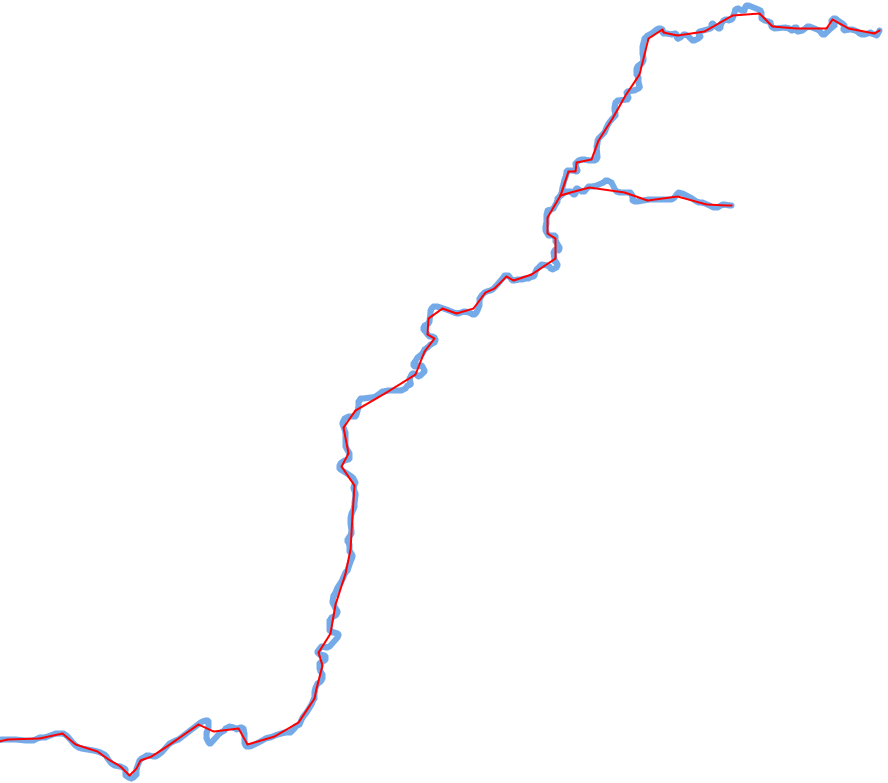# Description of Raposo Hexagon Based Line Simplification Algorithm

• Date 20/07/2017.
• Author: Guillaume Touya
• Contact {firstname.lastname}@ign.fr.

## Description of the algorithm

This algorithm simplifies lines based on a hexagonal tessallation, and is described in (Raposo 2013). The algorithm also works for the simplification of the border of a polygon object.

The idea of the algorithm is to put a hexagonal tessallation on top of the line to simplify, the size of the cells depending on the targeted granularity of the line. Similarly to the Li-Openshaw algorithm, only one vertex is kept inside each cell. This point can be the centroid of the removed vertices, or a projection on the initial line of this centroid. The shapes obtained with this algorithm are less sharp than the ones obtained with other algorithms such as Douglas-Peucker.

Parameter name Description Type Default value
use_method_1 if true, uses the center of the hexagonal cells as new vertex, if false, the center is projected on the nearest point in the initial line boolean true
use_tobler_resolution compute cell resolution based on Tobler’s formula if true, Raposo’s formula if false boolean true
initial_scale the initial scale of the data (25000.0 for 1:25000 scale) double

Tobler based formula to compute hexagonal cell size: cell_size = 5 x l x s where l is the width of the line in the map in meters e.g. 0.0005 for 0.5 mm, and s is the target scale denominator.

Raposo’s formula to compute hexagonal cell size: cell_size = l / n x t / d where l is the length of the line, n the number of vertices of the line, t the denominator of the target scale, and d the denominator of the initial scale

## Examples of generalization## When to use the algorithm?

The algorithm is dedicated to the smooth simplification of natural features such as rivers, forests, coastlines, lakes.